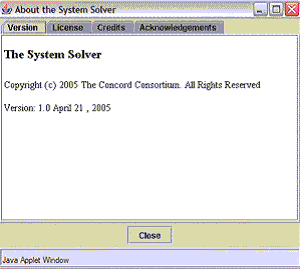# System Solver: User's Guide

The System Solver was developed to support you and your students' understanding of systems of linear equations. The System Solver offers users the unique opportunity to perform operations on a system and immediately observe the graphic, tabular, and symbolic results of the procedure at each step. Using the System Solver, you can:

• Select symbolic forms for different equations
• See the graphic results of operations on individual equations and systems
• See the graphic consequences of combining equations
• View the relationship between tabular results and graphic representations

Getting to Know the System Entry Interface

Entering a System

The Main System Solver Interface

Working with the Graph Window

Working with the Coordinate Table

Performing an Operation on Both Sides of the System

Combining Equations

Deleting and Starting Over

# Getting to Know the System Entry Interface

When you start the System Solver, the first screen is the system entry interface shown below. The interface contains two equation entry dialog boxes. This is the place where you select your equation form and enter the equations for the system you wish to solve. Each of these areas is explained in detail in this guide.System Entry Interface

As you go through this material, it's convenient to open the System Solver in a separate window so that you can work with the tool while following the instructions. Click System Solver to launch the interactive. When the System Solver launches, you will see the default values shown above.

Note If you click the Start Solving button immediately, before entering values, you will get a message indicating that the system that you have submitted is a dependent system. If at any time you find you have entered a system that gives you an error message, you can click on the Start Over button to return to the system entry interface (see Deleting and Starting Over below).

## Entering a System

In order to show you how the System Solver works, you'll be guided through a typical system solution that demonstrates the functionality of each part of the System Solver. Start with the following system.1. To select an appropriate equation format, click the Equation Format Selector and view the four equation formats to chose from. These should accommodate most of the system formats that you will encounter.

Note Although the illustrations and examples are shown in the dialog labeled Equation 1, the operation is identical for Equation 2.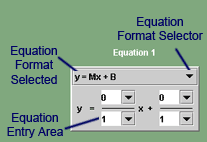Equation Entry Dialog Box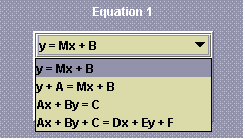Equation Format Selections
2. For our example, select the second format for Equation 1. Once you have selected the format, it appears at the top of the dialog.
3. Enter the values for Equation 1 into the Equation Entry Area. You must enter a numerator as well as a denominator for each value.
4. Select a format in the dialog labeled Equation 2, and enter values into the Entry fields.
5. You should now have a set of dialogs like the illustration below. Click the Start Solving button to accept your entries and advance to the main System Solver interface.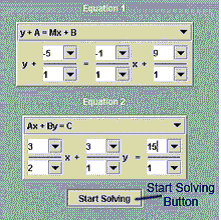Equation Entry Dialog Boxes

# the Main System Solver interface

Now that you have entered a system and clicked on the Start Solving button, the System Solver presents the main interface. Take a moment to note each part of the interface.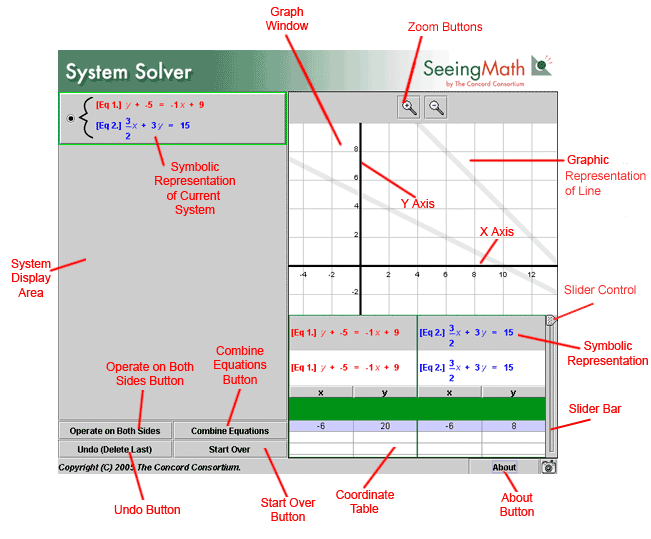Main System Solver Interface

## Working with the Graph Window

The main Graph Window contains a Cartesian plane showing a gray line on the x-axis and the y-axis for each equation in your system. There are also tools that allow you to modify the display. At the top of the screen are the Zoom buttons.Zoom Buttons

1. Click the magnifying icon with the plus sign to "zoom in."
2. Click the magnifying glass icon with the minus sign to "zoom out" and see a greater area.

As shown below, when you zoom out, you see a larger area of the plane. A blue and a red dot correspond to the colors of the equations below the Graph Window. The red dot is on the line represented by Equation 1. The blue dot is on the line that represents Equation 2. In this example, they share a common x-coordinate.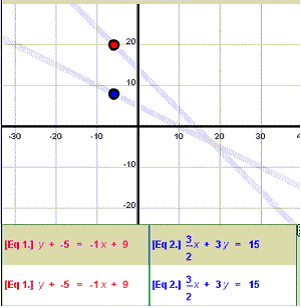A Shared x-Coordinate in the Graph Window

In the Coordinate Table (see below), you can see the shared x-coordinate is −6.

Note Scroll across the Graph Window by moving your cursor over the window until the cursor changes its shape to a hand. Then, click and hold while moving the Graph Window to reveal additional area.

# Working with the Coordinate Table

Below the Graph Window is the Tabular Area of the interface.This is where your two current system equations are displayed in the Symbolic Representation area. There is also a Coordinate Table with a row of numbers and a Slider Bar for displaying more values. The row of numbers represents the coordinates of the red and blue indicators in the Graph Window.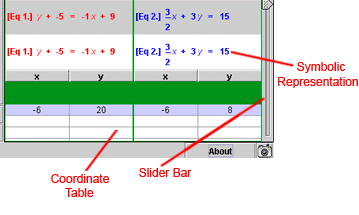Tabular Area

1. Click and hold the wedge-shaped Slide Control on the Slider Bar and move it up and down. You will see that three things happen.

• In the Graph Window, if you have maintained the earlier position, a series of additional red and blue coordinate indicators appear moving along the length of the lines, including an intersection point.
• In the Coordinate Table, you see coordinate sets that each correspond to one pair of red and blue indicators on the Graph Window.
• The lower set of Symbolic Representations of each equation change in a manner corresponding with the highlighted line on the Coordinate Table.
2. Move your mouse and click on any of the coordinate indicators in the Graph Window.

• The corresponding line of coordinates in the Coordinate Table highlight.
• The Symbolic Representations change to match.
3. Click on a line of coordinates in the Coordinate Table.

• Corresponding coordinate indicators highlight.
• Note that one set of coordinates in the table is in bold text. That line represents the coordinates where the two lines intersect. The Symbolic Representations change to match.

# Performing an Operation on Both Sides of the System

The traditional way to find the intersection of a system is through algebraic operations. The System Solver allows you to perform an operation on both sides of an equation within a system, using dialog boxes.

1. Click on the Operate on Both Sides button to bring up the Term Operations Dialog Box.Operation Selection ButtonsTerm Operations Dialog Box
2. In the Equation Selection Area, select which equation in the system you want to perform the operation on by clicking the corresponding radio button to the left.

• As you can see in the illustration above, Equation 1 has been selected.
• In the Operation section, the Operation Selector allows you to choose which type of operation to perform on the selected equation.Operation Selections

3. In this example, select "Add to each side" as the operation to perform on Equation 1.
4. In the Values Entry Area, enter the whole number 5.
• Since you must use fractions, enter the number 5 in the numerator. Make sure the denominator is 1. You can also use the drop-down arrows next to the Values Entry Area to select your entries. Doing so permits the Real Time Results section at the bottom of the dialog to preview your results. This allows you to experiment with several different possibilities before making a final operation decision.
• To the right is the (x, y) Selector. If you were performing the operation on one of the variables, you would have selected it there.
5. After entering the values and choosing "Add to each side," click on the Accept button at the bottom of the dialog.

• This will close the dialog and return you to the main System Solver screen where you now see a new view of your system containing the original Equation 2 and a new representation of Equation 1 called Equation 3. (Clicking the cancel button also allows you to return to the main System Solver screen but without committing any changes to the system.)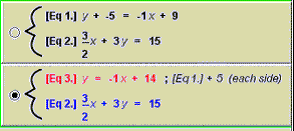Result of First Operation

6. The resulting system retains the colors of the original equations, with Equation 1 and Equation 3 remaining red. Notice that the equations represented in the Tabular Area also change to reflect the currently selected system representation. Clicking the radio button next to the original set returns the color (and operational) focus to them and changes the Tabular Area representation as well.

• Returning the focus to the second representation of the system shows that our operation has now successfully defined y in terms of x by isolating y on one side of Equation 3. A note to the right of the equation reminds us how we achieved that result.

# Combining Equations

Next, you will perform a function operation on the selected system, substituting Equation 3 into Equation 2.

1. With the system of Equation 3 and Equation 2 selected, click on the Combine Equations button.Operation Selection Buttons

This brings up the Function Operations Dialog.Function Operations Dialog

2. The Equation Selectors allow you to choose which equation will operate on the other. In this example, we want to reverse the default order shown and have Equation 3 operate on Equation 2. To do so, click on the Equation Selectors and choose the appropriate equations from the resulting menu.

Note that as you do so, the results in the Real Time Results section change to show how your operation will be reflected in a new equation, Equation 4.

3. The default operation is Add. In this case, we want you to Substitute Equation 3 into Equation 2 rather than add. Click on the Operation Selector to show the types of operations you can apply. Scroll down and click on Substitute.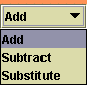Function Operations Selections

Note that when you choose Substitute, the new Equation 4, in the Real Time Results area, changes to reflect the result of the operation.

4. Click the Accept button to accept the operation. You should now see results in your System Display Area.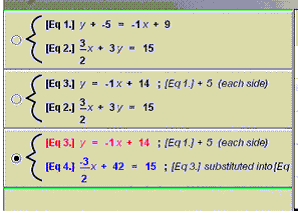Result of Second Operation

5. If you now continue by subtracting 42 from both sides of Equation 4, dividing each side of the resulting Equation 5 by -2/3, and then substituting Equation 6 into Equation 3, you will have solved the system and have a System Display Area that looks like the following illustration.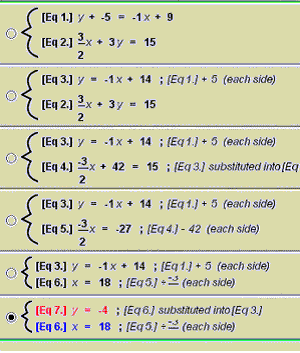System Display Area Showing Solved System

You have solved the system and found that the coordinates of (y = −4) and (x = 18) are the intersection point of the system.

# Deleting and Starting Over

The last two buttons of interest in the Symbolic Representation Area are the Undo button and the Start Over button.Undo Button and Start Over Button

• Clicking on the Undo button takes you back one step in your solving process.
• Clicking on the Start Over button ends your current system session and returns you to the System Entry Interface where you can enter a new system.

Note You can't delete your initial system using the Undo button. If you wish to delete that system, use the Start Over button instead.